双重标准？ Retina屏科学原理

年薪达不到25W大数据工程师、拿不到Offer全额退款->>>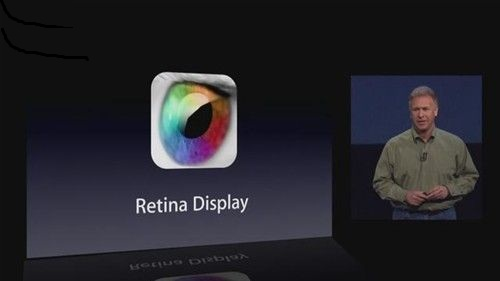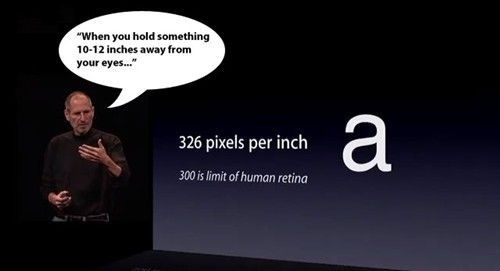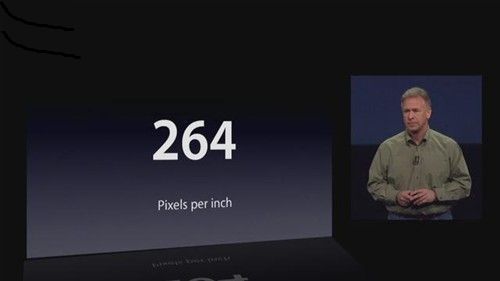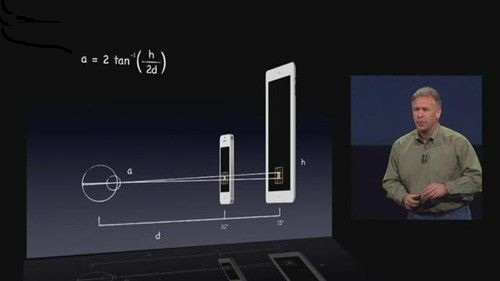α＝2tan-1（h/2d）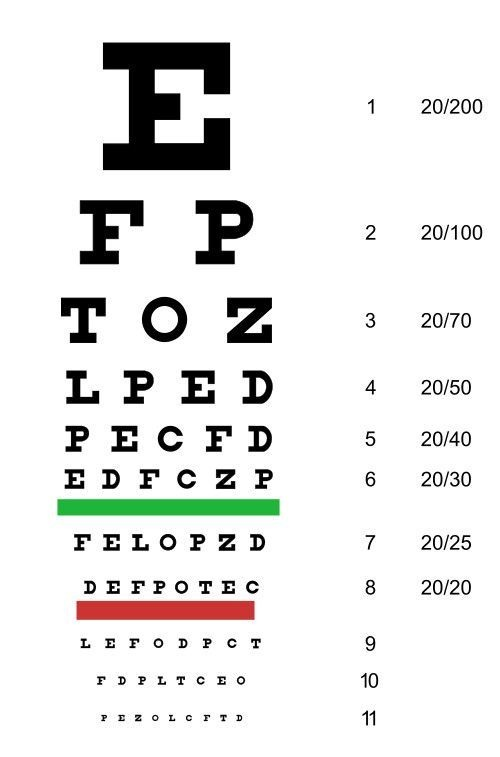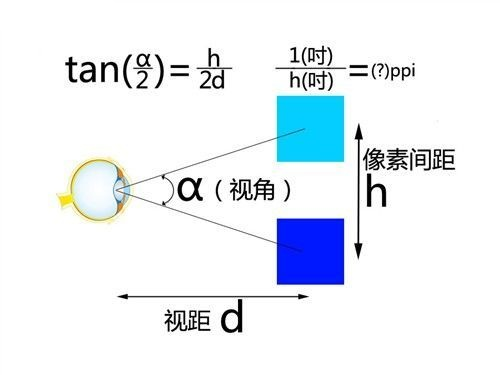也就是说，是否为 Retina 屏幕，不仅仅决定于 ppi（分辨率，或者说像素间距h；1英寸/像素间距h英寸＝ppi），还要看使用设备时与人眼的距离（d）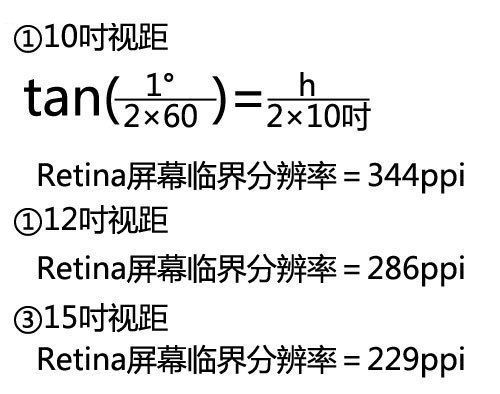1） 10寸

h = tan(1/(2x60)) x (2x10) = tan(1/120) x 20 = tan(0.008333333) x 20 = 0.000145444 x 20 = 0.00290888

ppi = 1英寸/像素间距h英寸 = 1/h = 1/0.00290888 = 343.774923682 = 344

2） 12寸

h = tan(1/(2x60)) x (2x12) = tan(0.008333333) x 24 = 0.003490656

ppi = 1英寸/像素间距h英寸 = 1/h = 1/0.003490656 = 286.479103068 = 286

3） 15寸

h = tan(1/(2x60)) x (2x15) = tan(0.008333333) x 30 = 0.00436332

ppi = 1英寸/像素间距h英寸 = 1/h = 1/0.00436332 = 229.183282455 = 229

1） iPhone4/4S 如果在10 吋（25.4 厘米）的距离上使用，并不是 Retina 屏（分辨率至少要达到 344ppi 才是，对于乔布斯“在 10-12 吋，300ppi是人类视觉极限”的说法，Phil Schiller 这是在打脸啊）；在11 吋（28 厘米）的距离上使用，略超 Retina 屏的标准。

2） 新 iPad 屏幕分辨率 264ppi，如果使用距离为 15 吋（约 38 厘米），超过了这个距离上 Retina 屏幕标准的 23％

Apple 当前主要retina屏幕计算方式：

ppi = （w x w + h x h）^ （1/2） / size  （其中， w是屏幕宽度， h是屏幕高度， size是屏幕对角线长度， 单位都为英尺 inch ）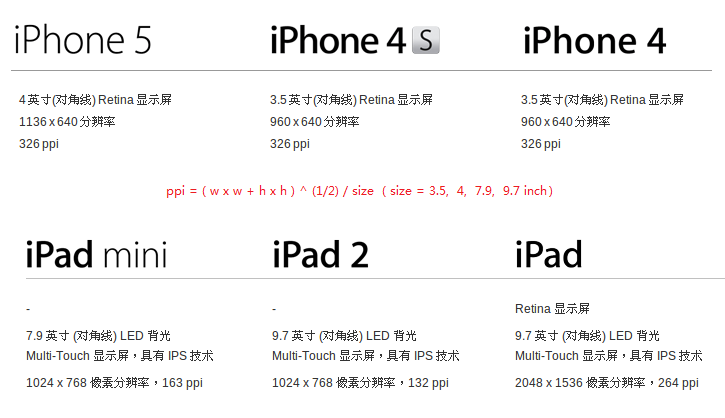1） iphone 5

ppi = （w x w + h x h）^ （1/2） / size = （1136 x 1136 + 640 x 640） ^ (1/2) / 4 = 1700096 ^ (1/2) / 4 = 1303.87729484 / 4 = 325.96932371 = 326

2） iphone 4

ppi = （w x w + h x h）^ （1/2） / size = （960 x 960 + 640 x 640） ^ (1/2) / 3.51331200 ^ (1/2) / 3.5 = 1153.776408148 / 3.5 = 329.650402328 = 330   (官方326不准确)

ppi = （w x w + h x h）^ （1/2） / size = （1024 x 1024 + 768 x 768） ^ (1/2) / 7.9 = 1638400 ^ (1/2) / 7.9 = 1280 / 7.9 = 162.025316456 = 162

4） iPad 4 （Retina）
ppi = （w x w + h x h）^ （1/2） / size = （2048 x 2048 + 1536 x 1536） ^ (1/2) / 9.7 = 6553600 ^ (1/2) / 9.7 = 2560 / 9.7 = 263.917525773 = 264

1米 = 3尺
1尺 = 10寸

1米 = 100厘米
30寸 = 100厘米（=1米）
1寸 = 100/30=3.3333333厘米
10 = 100/30x10=33.333333厘米